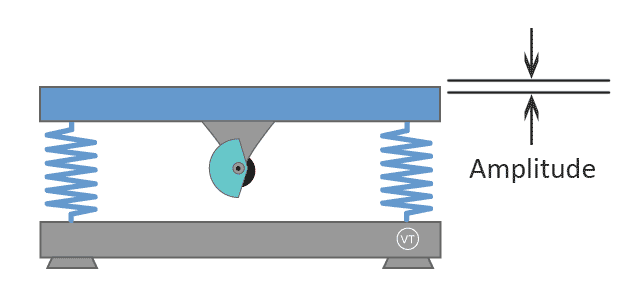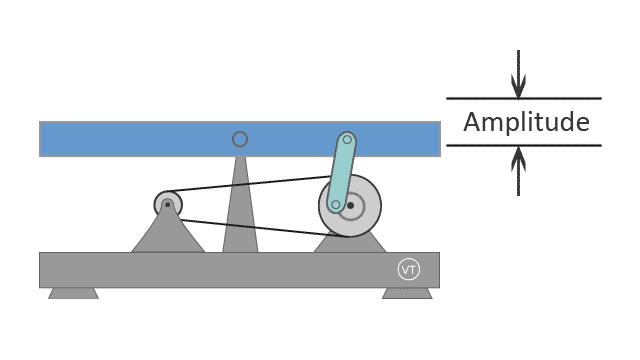devoted to
making better vibration plates# Terminologies of Vibration plate

• Vibration Frequency
• Speed Level
• Vibration Amplitude
• Vibration G-force
• ## Vibration Frequency

Vibration frequency indicates how many reciprocating movements occur each second. Its unit is hertz (Hz).

[Example] A vibration plate running at 30Hz means that it moves up-and-down 30 times in each second.

The animations below demonstrate the rotation speed of a linear vibration running at 5Hz in comparison with running at 10Hz.Frequency = 5Hz Upper plate moves up-and-down 5 times in a second. Frequency = 10Hz Upper plate moves up-and-down 10 times in a second. Linear vibration plates are usually designed to run at a high frequency range from 15Hz to 40Hz

The animations below demonstrate the rotation speed of a pivotal oscillation plate running at 5Hz in comparison with running at 10Hz.Frequency = 5Hz Upper plate moves up-and-down 5 times in a second. Frequency = 10Hz Upper plate moves up-and-down 10 times in a second. Pivotal oscillation plates are usually designed to run at a low frequency range from 5Hz to 14Hz

Some vibration plate models are designed to run at a fixed frequency. Some vibration plate models are designed to run adjustable frequency.

## Speed Level

Speed level is NOT a standard term to describe vibration. However many manufacturers use Speed Level on their vibration plates.

Each manufacturer defines speed level differently. You cannot compare speed level from model to model. A model with 99 speed level does not mean it is fast than another model with 20 speed level.

Example

LifePro Waver is a pivotal oscillation plate sold by LifePro. Its speed level is configured to range from 1 to 99 to control its adjustable frequency from 4Hz to 12Hz. At the speed 99, its vibration is at 12Hz.

VT027 is a pivotal oscillation plate made by Vibration Therapeutic. Its speed level is configured to range from 1 to 20 to control its adjustable frequency from 5Hz to 14Hz. At speed 20, its vibration is at 14Hz.

This example demonstrates a machine with 99 speed level is actually slower than a machine with 20 speed level.

Some manufacturers configure their low frequency vibration plate to have 180 speed level. This actually make the machine very awkward to use. It is only for a marketing hype.

Hz is the standard term to describe how fast the vibration runs.

## Vibration Amplitude

Vibration amplitude is the maximum vertical travel distance of the upper platform of a vibration plate.

For example, a linear plate has an amplitude of 3mm. This means the distance between the highest position and the lowest position of is upper platform is 3mm.

Linear vibration plates are usually designed with a lower amplitude, mostly less than 3mm.In physics, the distance between the highest position and the lowest position is called peak-to-peak amplitude, which is twice the value of amplitude referred in physics.

Some linear vibration plate models are designed to have two amplitude settings, e.g. model VT003F and VT007 by Vibration Therapeutic®

Pivotal oscillation plates are usually designed to produce higher amplitudes, usually in a range between 8mm and 10mm.Due to its see-saw motion pattern, the amplitude at the center pivotal axis is zero, and towards the edges of the platform, the amplitude reaches its maximum.

Pivotal oscillation plates do not have an adjustable amplitude setting.

## Vibration G-force

G-force is an important indicator of vibration intensity. Some manufacturers don't provide the G-force value of their vibration plates, but if you know the frequency and the amplitude, you can calculate the G-force of your machine.

So called G-force, it is actually not a force, but an acceleration rate expressed in the unit of G, the acceleration rate of gravity.

G-force provides a good way to compare the intensity of different vibration plates.